Elliptic-curve Cryptography, IoT Security, and Cryptocurrencies
Posted by Alina 10 months agoThe Famous Edwards Curves

Feb 16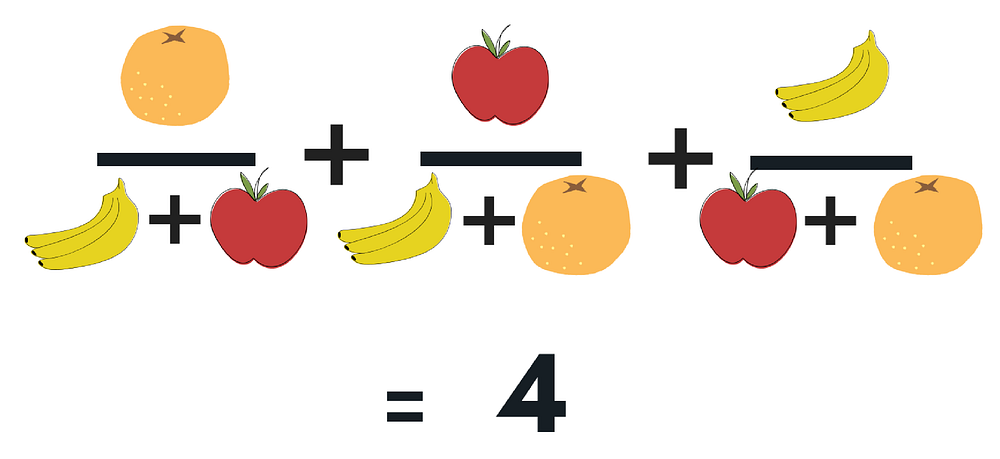Bet you thought that this puzzle is for kids! Actually, this brain teaser requires a tad more than simple math. Yesterday, we shared a similar brain teaser that is slightly easier with the rest of the IoTeX community.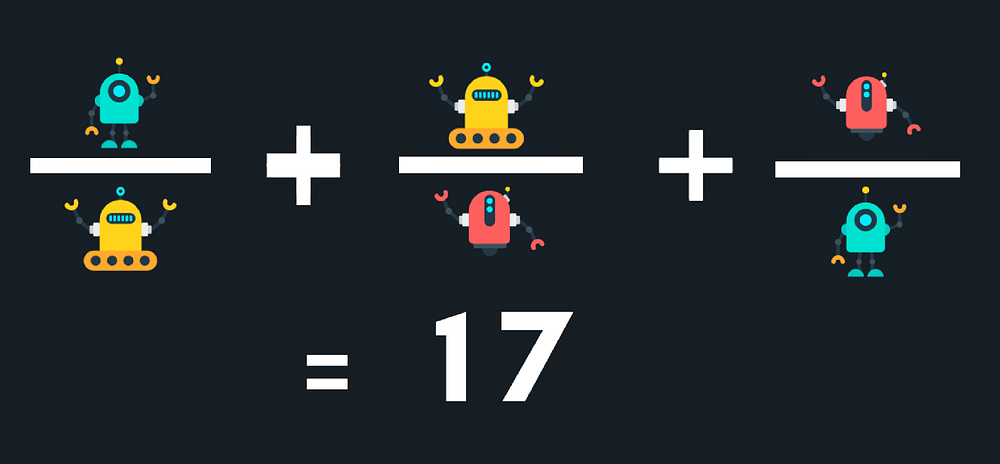Within three hours, we had our first winner! So far we have 45 people who have answered the brain teaser correctly, so keep trying! This was not an easy feat! IoTeX community is full of genius level pros.

Don’t worry if you did not manage to solve the above brain teaser. The IoTeX team has a bunch of upcoming bounty events for you. Tune in for updates!

Now that we have gotten the announcement out of the way. Let’s get down to business. For this week’s tech space, our developers were playing around with the original brain teaser and our team decided to write a short crash course on elliptic-curve cryptography (ECC).

Mini Elliptic-curve Crash Course

Elliptic-curve cryptography (ECC) is what IoTeX used to build our blockchain platform. Its primary purpose is to protect blockchain transactions, maximizing security and privacy for all DApps hosted on our blockchain platform. Note: We do not encrypt the blockchain transactions. Instead we hide transaction values using a commitment scheme.

Disclaimer: This blogpost is meant to cover the basics of ECC. This is not a udemy course on everything you need to know about ECC.

Remember your algebra from college?

Group (G, +)Ring (R, +, ×) and Field (F, +, ×).

Note that G, R and F are defined as different elements and one can perform various operations with these elements (see Table1). To simplify things, you may think of the operations “+”, “-”, “×” and “÷” as addition, subtraction, multiplication, and division, respectively.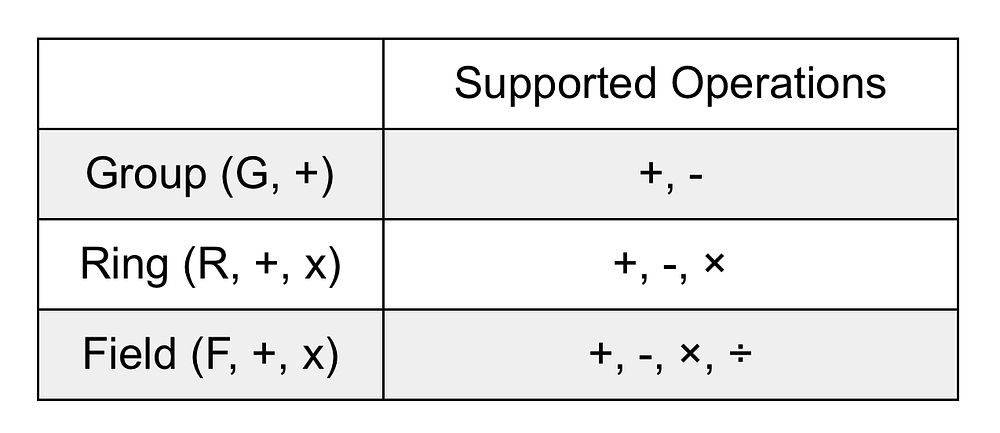Table 1. Supported Operations in Group, Ring and Field

If you have never seen an elliptic-curve before, it is nothing but a group (E, ⊕) defined over an underlying field (F, +, x). Since E is a group, you can perform the operations “⊕” and “⊖” over E. Operations in the underlying field F (i.e., +, -, ×, ÷) are used and combined to compute the elliptic-curve group operations “⊕” and “⊖”.

Are you lost yet?

Let’s go through a simple example together and explore what group (E, ⊕) should look like. To this end, we consider an elliptic-curve E defined over a prime field F11 as follows:

E/F11: y^2 = x^3 + 4x + 3,

where F11 = {0, 1, … ,10} is a prime field with 11 elements. In order to find the solutions for the above elliptic-curve equation, we first need to generate an addition and multiplication tables over F11: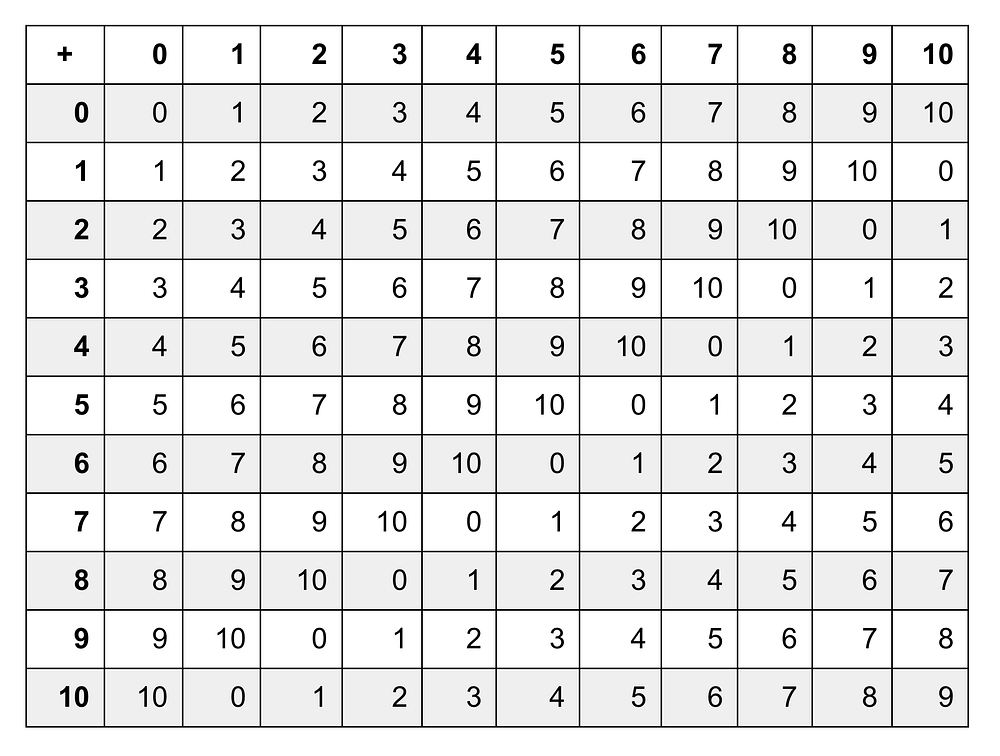Table 2. Addition over F11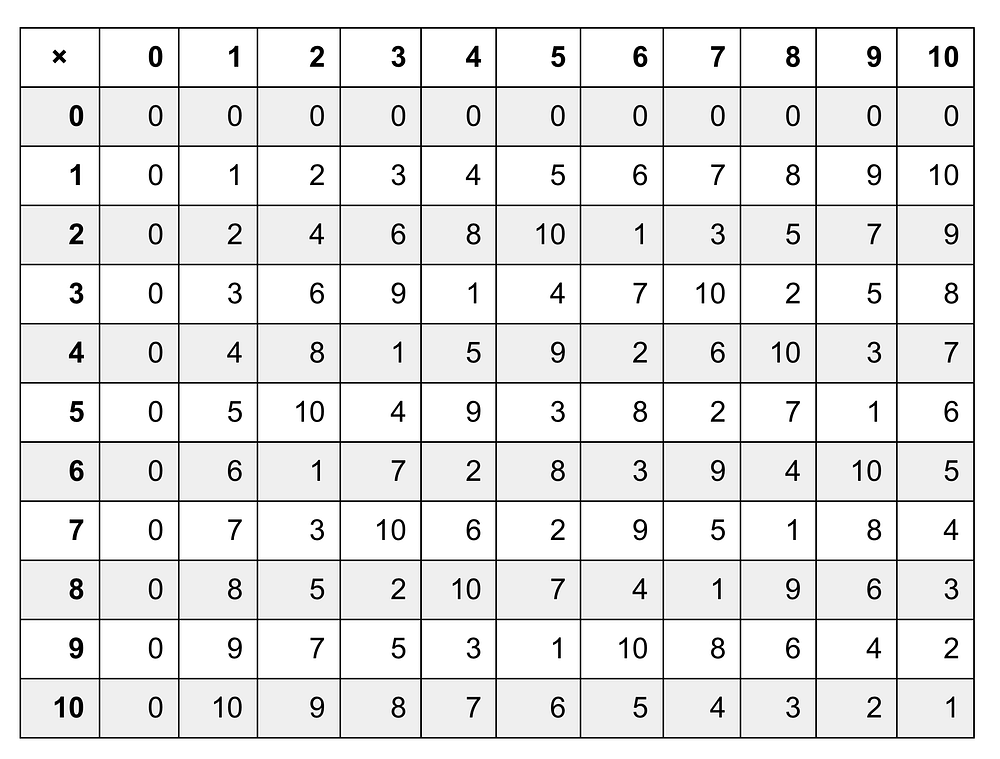Table 3. Multiplication over F11

By conducting an exhaustive search, you can find 13 solutions over F11 for the above equation, namely (0, 5), (0, 6), (3, 3), (3, 8), (5, 4), (5, 7), (6, 1), (6, 10), (7, 0), (9, 3), (9, 8), (10, 3) and (10, 8), as shown in the figure below.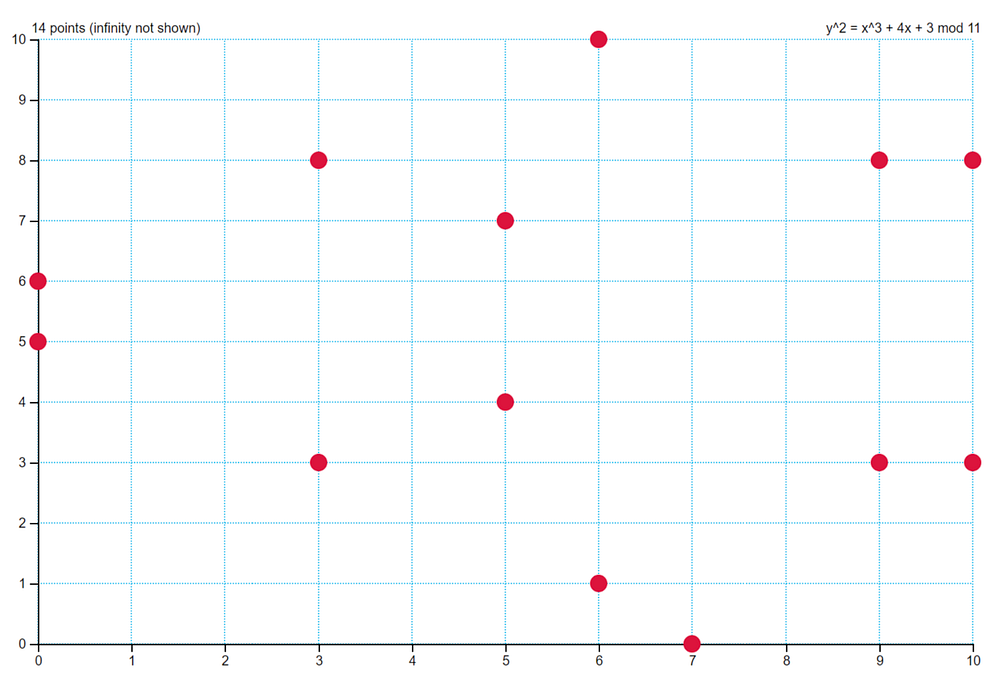All of these 13 solutions and a special point O (so-called point at infinity) form a group of 14 elements, i.e., E(F11) = {(0, 5), (0, 6), (3, 3), (3, 8), (5, 4), (5, 7), (6, 1), (6, 10), (7, 0), (9, 3), (9, 8), (10, 3), (10, 8), O}. Now that we have the group E(F11), it is time to talk about the operations “⊕” and “⊖” in this group. Given the following two points P and Q in E(F11), how can we get the third point R in this group so that P⊕Q = R? The answer is to follow the so-called “chord-and-tangent” rule as illustrated in the figure below: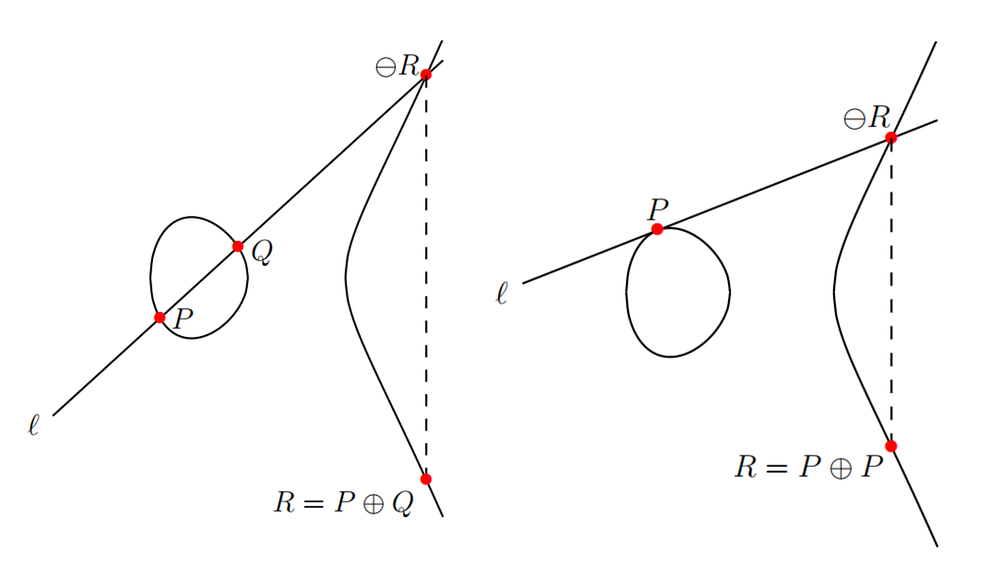According to this rule, if you want to compute P⊕Q (resp. P⊕P), you can draw a chord (resp. tangent) line through those two (resp. one) points, which intersects with the elliptic-curve at the point ⊖R. You then flip this point over x-axis to get the point R = P⊕Q (resp. R = P⊕P). Mathematically speaking, the rules for adding two points P and Q over an elliptic-curve E(F11) are defined as follows:

1. P⊕= P, where is the point-at-infinity.
2. If P = (Xp, Yp), then P⊕(Xp, -Yp) = O. The point (Xp, -Yp) is the negative of P, denoted by ⊖P. For example, in E(F11) if P = (5, 4), then ⊖P = (5, -4) = (5, 7) since -4 ≡ 7 mod 11, which is also in E(F11).
3. If P = (Xp, Yp) and Q = (Xq, Yq) with P ≠ ⊖Q, then R = P⊕Q = (Xr, Yr) is in E(F11) and determined by the following formulae: Xr = (λ^2 — Xp — Xq) mod 11 and Yr = [λ(Xp — Xr) — Yp] mod 11, where λ = (Yp — Yq) / (Xp — Xq) mod 11, if P ≠ Q. In the case of P = Q, λ = (3Xp^2 + 4) / 2Yp mod 11.
4. Multiplication by an integer k is defined by repeated addition, which is usually called a scalar multiplication. For example, 5P = P⊕P⊕P⊕P⊕P.

With these simple formulae, you can play around with E(F11) and jump from one point to the other. You should know how to solve our teaser by now, right?

Elliptic-curve cryptography

Any public key cryptosystem is based on difficult computational problems. Elliptic-curve cryptosystem is no exception. Consider the equation Q = kP, where P and Q are two points over an elliptic-curve E(Fp) with p being a sufficiently large prime (e.g., ~2^160). While it is relatively easy to calculate Q given k and P, it is extremely difficult to determine k given Q and P. This is called elliptic-curve discrete logarithm problem (ECDLP). From our simple exercise E(F11) above, the discrete logarithm problem can be easily solved via a table-lookup. Given that P = (3, 3), we can compute kP, k = 0, …, 14, using the point addition formulae and put the results in a table as follows: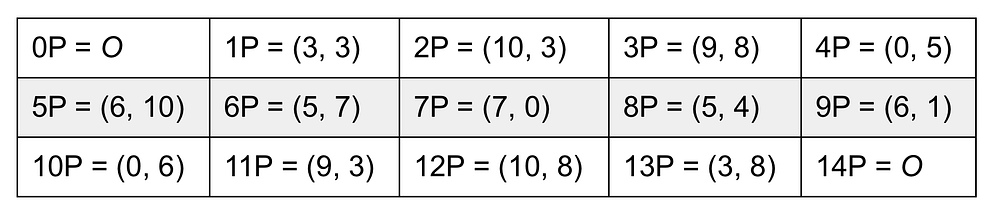Table 4.

Given any Q in E(F11), we can find the corresponding k such that Q = kP. For example, for Q = (5, 4), we know that k = 8 by checking the above table. While this method works well for our simple exercise, it is not applicable when p is a large prime. Therefore, the ECDLP ensures the security of elliptic-curve cryptosystems in practice. Various useful security protocols, such as Elliptic-Curve Diffie-Hellman (ECDH) key exchange protocolElliptic-Curve Digital Signature Algorithm (ECDSA), etc., can be designed using a similar approach.

Elliptic-curve Cryptography for IoT Security and Cryptocurrencies

ECC offers the same security level when you compare it with RSA. A few advantages of ECC are that it has much shorter operand size and more efficient implementations. Over the years, it has become a de-facto standard for protecting security and privacy for emerging IoT systems and cryptocurrency networks. Multiple national and international organizations have standardized the use of elliptic-curves in cryptography. In particular, National Institute of Science and Technology (NIST) has standardized ECC for digital signature algorithms in FIPS 186 and key establishment schemes in NIST special Publication 800–56A. In FIPS 186–2, NIST recommended 15 elliptic-curves of different security levels for use in ECC standards. Moreover, the Standards for Efficient Cryptography Group (SECG), which is an international consortium founded by Certicom in 1998, has developed commercial standards for efficient and interoperable cryptography based on ECC. In “SEC 2: Recommended Elliptic-curve Domain Parameters”, SECG suggested 33 elliptic-curves of various security levels for use in practice, including the 15 elliptic-curves recommended by NIST.

In the IoT security and cryptocurrency domains, ECC has become a popular choice among the IoT device manufactures, system architects and standardization bodies protecting resource-constrained smart environments such as ZigBee Smart Energy, Vehicular Ad-Hoc Networks, Near-field Communications (NFC), etc., as well as sensitive transactions in cryptocurrencies.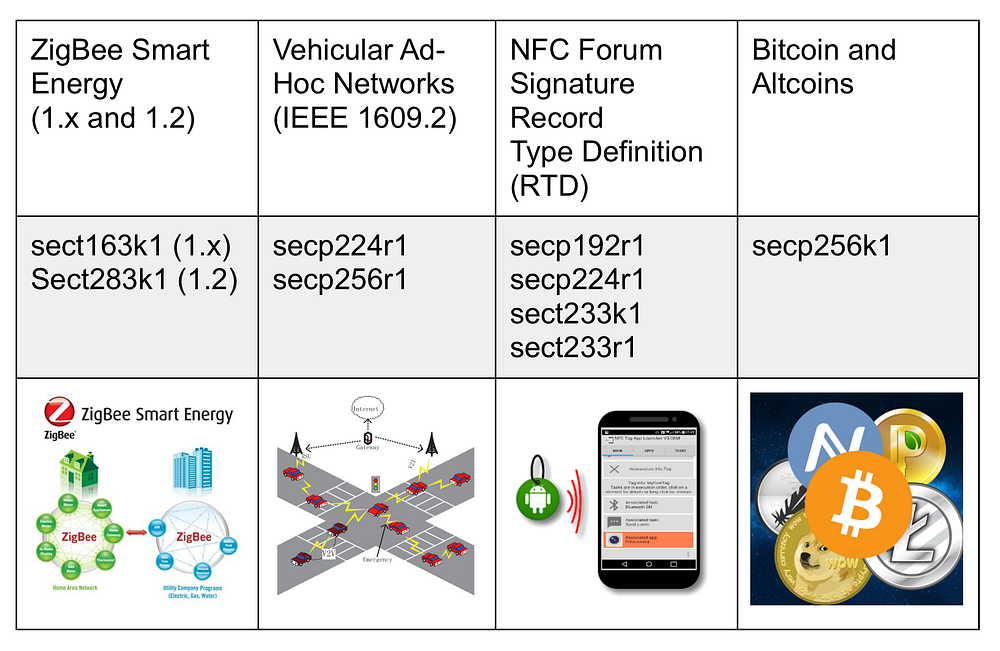Table 5. Elliptic-curves Used in Various Applications.

Note: The elliptic-curve nicknames are taken from “SEC 2: Recommended Elliptic-curve Domain Parameters”.

Brave New Curves

It has been more than 15 years since ECC was standardized by NIST and SECG. The crypto research community and industry practitioners have gained much deeper understanding with regards to ECC security and implementation issues in the recent years. As a result, multiple new elliptic-curves, which mainly target 128-bit or higher security level, have been proposed in the literature.

Some interesting examples:

• Curve25519: It was proposed by Daniel J. Bernstein in 2005. This elliptic-curve offers 128-bit security and was designed to use with elliptic-curve Diffie-Hellman (ECDH) key exchange protocol. Curve25519 has been adopted by popular messaging apps such as WhatsApp and Signal, among others.
• FourQ: It was proposed by researchers from Microsoft Research in 2015 and offers 128-bit security. FourQ claims to be ~4 to 5 times faster than NIST P-256 curve and ~2 to 3 times faster than Curve25519.
• GLS254: It was proposed by a group of researchers from Cinvestav-IPN and University of Campinas in 2013 and offers 127-bit security. GLS254 is a binary elliptic-curve and it has the fastest implementation among all new curves.

All of these new curves have been peer-reviewed by the crypto community and achieved high performance across modern implementation platforms in practice. If you are designing a new security application and interoperability is not your top priority, then you may consider trying out these curves.

ECC for IoTeX Blockchain

Like many other blockchain platforms, the security and privacy of transactions on the IoTeX blockchains are also protected by ECC. Our developers, however, have two more thing to worry about: heterogeneity of the IoT devices and interoperability among them. Our goal is to get the various IoT applications and machine-to-machine communications to be seamless. IoTeX’s testnet implements a binary Koblitz curve sect283k1, which is adopted by ZigBee Alliance for powering smart energy and smart home applications. Other elliptic-curves will be added into the IoTeX crypto core in the future for supporting a wide range of IoT applications.

178 Views0 Replies0 Subscriptions